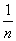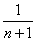# Statistics Assignment Help With Discrete Uniform Distribution

## 5.8 Discrete Uniform Distribution

A random variable X is said to have uniform distribution on n points {x1,x2,……..,xn} if its p.m.f. is given by:

P(X=xi) =i=1,2,……….,nFor example, if X has a uniform distribution on the points { 0,1,2,…,n}, then P(X =i) =i=0,1,2,…..,n

Such distributions can be concelved in practice if under the given experimental conditions, the different values of the random variable become equally likely. Thus for a die experiment, and for an experiment with a deck of cards such distribution is appropriate.